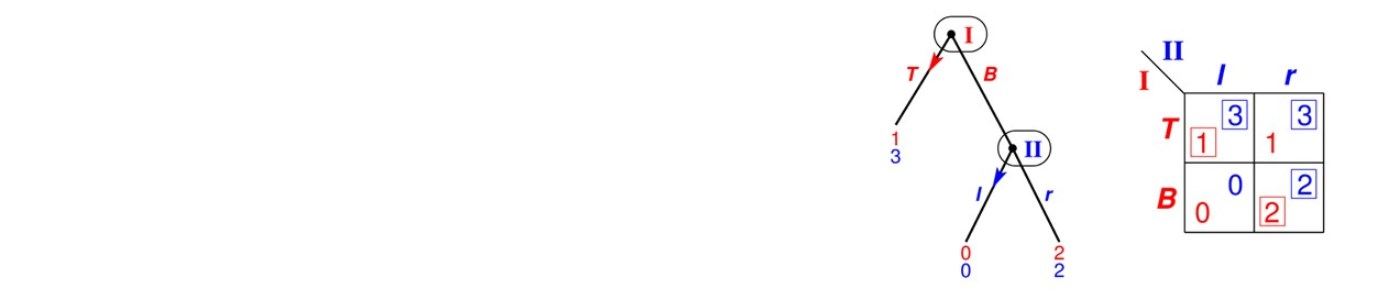# Game Theory Research

Game theory is the formal study of conflict and cooperation. Game theoretic concepts apply whenever the actions of several agents are interdependent. These agents may be individuals, groups, firms, or any combination of these. The concepts of game theory provide a language to formulate, structure, analyse and understand strategic scenarios.

Individual faculty interests are listed below.

## A

Dr Galit Ashkenazi-Golan

• Repeated games
• Markov decision processes and Stochastic games
• Borel games
• Games with incomplete information
• Games with imperfect monitoring

## G

Professor Olivier Gossner

• Economics of information
• Bounded rationality and complexity
• Repeated games
• Game theory in general

## L

Professor Andrew Lewis-Pye

• Algorithmic processes
• Randomness
• Computability
• Algorithmic game theory
• Agent based models
• Networks
• Discrete mathematics in general

## S

Dr Robert Simon

• Measure theory
• Ergodic theory
• Functional analysis
• Stochastic processes
• Chaos Theory
• Combinatorics
• Algebraic topology

Professor Bernhard von Stengel

• Game theory: efficient computation of equilibria, theory of online algorithms
• Extensive form games
• Correlated equilibria
• Pivoting algorithms in linear programming and linear complementarity problems
• Polytope theory

## W

Dr Nicola Wittur

• Game theory
• Information theory